# Problem: This problem illustrates the two contributions to the kinetic energy of an extended object: rotational kinetic energy and translational kinetic energy. You are to find the total kinetic energy  of a dumbbell of mass when it is rotating with angular speed and its center of mass is moving translationally with speed .  Denote the dumbbell's moment of inertia about its center of mass by . Note that if you approximate the spheres as point masses of mass  each located a distance  from the center and ignore the moment of inertia of the connecting rod, then the moment of inertia of the dumbbell is given by , but this fact will not be necessary for this problem. Find the total kinetic energy of the dumbbell. Express your answer in terms of m, v, , and .

###### FREE Expert Solution

Translational kinetic energy:

$\overline{){\mathbf{K}}{{\mathbf{E}}}_{{\mathbf{T}}}{\mathbf{=}}\frac{\mathbf{1}}{\mathbf{2}}{\mathbf{m}}{{\mathbf{v}}}^{{\mathbf{2}}}}$

Rotational kinetic energy:

$\overline{){{\mathbf{KE}}}_{{\mathbf{R}}}{\mathbf{=}}\frac{\mathbf{1}}{\mathbf{2}}{{\mathbit{I}}}_{\mathbf{c}\mathbf{m}}{{\mathbit{\omega }}}^{{\mathbf{2}}}}$

Total kinetic energy:

$\overline{){\mathbf{KE}}{\mathbf{=}}{\mathbit{K}}{{\mathbit{E}}}_{{\mathbf{T}}}{\mathbf{+}}{\mathbit{K}}{{\mathbit{E}}}_{{\mathbf{R}}}}$

The total kinetic energy:

88% (82 ratings)###### Problem Details

This problem illustrates the two contributions to the kinetic energy of an extended object: rotational kinetic energy and translational kinetic energy. You are to find the total kinetic energy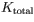of a dumbbell of mass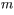when it is rotating with angular speed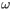and its center of mass is moving translationally with speed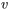.

Denote the dumbbell's moment of inertia about its center of mass by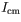. Note that if you approximate the spheres as point masses of mass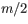each located a distance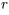from the center and ignore the moment of inertia of the connecting rod, then the moment of inertia of the dumbbell is given by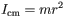, but this fact will not be necessary for this problem. Find the total kinetic energy of the dumbbell. Express your answer in terms of m, v,, and.

Frequently Asked Questions

What scientific concept do you need to know in order to solve this problem?

Our tutors have indicated that to solve this problem you will need to apply the Intro to Rotational Kinetic Energy concept. You can view video lessons to learn Intro to Rotational Kinetic Energy. Or if you need more Intro to Rotational Kinetic Energy practice, you can also practice Intro to Rotational Kinetic Energy practice problems.